## RS Aggarwal Class 6 Solutions Chapter 14 Constructions (Using Ruler and a Pairs of Compasses) Ex 14A

These Solutions are part of RS Aggarwal Solutions Class 6. Here we have given RS Aggarwal Solutions Class 6 Chapter 14 Constructions (Using Ruler and a Pairs of Compasses) Ex 14A.

Other Exercises

Question 1.
Solution:
Steps of construction :
(i) Draw a line segment PQ = 6.2 cm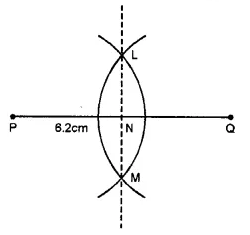(ii) With centre P and Q and radius more than half of PQ, draw arcs on each side intersecting each other at L and M.
(iii) Join LM intersecting PQ at N.
Then, LM is the perpendicular bisector of PQ.

Question 2.
Solution:
Steps of Construction :
1. Draw a line segment AB = 5.6 cm.
2. With A as centre and radius more than half AB, draw arcs, one one each side of AB.3. With B as centre and same radius as before, draw arcs, cutting the previous arcs at P and Q respectively.
4. Join P and Q, meeting AB at M. Then PQ is the required perpendicular bisector of AB.
Verification : Measure ∠AMP. We see that ∠AMP = 90°. So, PQ is the perpendicular bisector of AB.

Question 3.
Solution:
Steps of Contruction :
1. Draw a ray RX.
2. With O as centre and any radius draw an arc cutting OA and OB at P and Q respectively.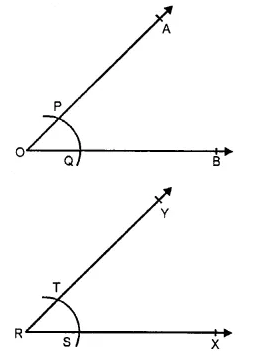3. With R as centre and same radius draw an arc cutting RX at S.
4. With S as centre and radius PQ cut the arc through S at T.
5. Join RT and produce it to Y. Then ∠XRY is the required angle equal to ∠AOB.
Verification: Measuring angle AOB and ∠XRY, we observe that ∠XRY = ∠AOB.

Question 4.
Solution:
Steps of constructions :
(i) Draw an angle ABC = 50° with the help of a protractor.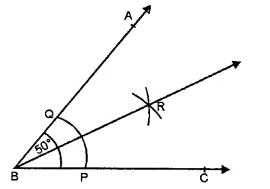(ii) With centre B and C and a suitable radius, draw an arc meeting AB at Q and BC at P.
(iii) With centres P and Q and with a suitable radius draw two arcs intersecting each other at R inside the angle ABC.
(iv) JoinRB.
Then ray BR is the bisector of ∠ABC.

Question 5.
Solution:
Steps of construction :
(i) Draw an angle AOB = 85° with the help of the protractor.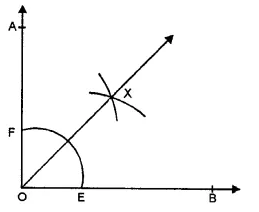(ii) With centre O, draw an arc with a suitable radius meeting OB at E and OA at F.
(iii) With centre E and F and with a suitable radius draw arcs intersecting each other at X inside the angle AOB.
Then ray OX is the bisector of ∠AOB.

Question 6.
Solution:
Steps of Construction :
(1) Draw the given line AB and take a point P on it.(2) With P as centre and any suitable radius draw a semi-circle to cut the line AB at X and Y.
(3) With centre X and radius more than XP draw an arc.
(4) With centre Y and same radius draw another arc to cut the previous arc at
(5) Join PQ. Then, PQ is the required line passing through P and perpendicular to AB.
Verification : Measure ∠APQ, we see that ∠APQ = 90°

Question 7.
Solution:
Steps of Construction :
(1) Draw the given line AB and take a point P outside it.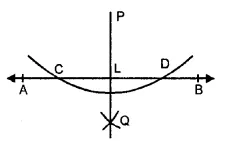(2) With P as centre and suitable radius, draw an arc intersecting AB at C and D.
(3) With C as centre and radius more than half CD, draw an arc.
(4) With D as centre and same radius, draw another arc to cut the previous arc at Q.
(5) Join PQ, meeting AB at L. Then PL is the required line passing through P and perpendicular to AB.
Verification : Measure ∠PLB. We see that ∠PLB = 90°.

Question 8.
Solution:
Steps of Construction :
1. Draw a given line AB and take a point P outside it.2. Take a point R on AB
3. Join PR.
4. Draw ∠RPQ such that ∠RPQ = ∠PRB as shown in the figure.
5. Produce PQ on both sides to form a line. Then, PQ is the required line passing through P and parallel to AB.
Verification: Since ∠RPQ = ∠PRB and these are alternate interior angles, it follows that PQ || AB.

Question 9.
Solution:
Steps of Construction :
1. Draw a ray BX and cut of BC = 5 cm.
2. With B as centre and suitable radius draw an arc above BX and cutting it at P.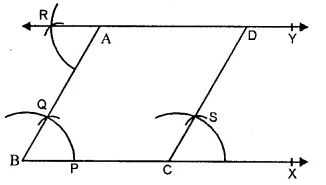3. With P as centre and the same radius as before draw another arc to cut the previous arc at Q.
4. Join PQ and produce it to the point A such that. AB = 4.5 cm. Then ∠ABC = 60° is the required angle.
5. Draw ∆RAB such that ∆RAB = ∆ABC.
6. Produce RA on both sides to form a line. Then, RY is the line parallel to BC and passing through A.
7. Now, draw ∆SCX = ∆ABC at the point C.
8. Produce CS to intersect the line RY at D.Then CD is the required line through C and parallel to AB.
9. Measure AB and CD. We see that AD = 5 cm. and CD = 4.5 cm.
Verification. Since ∠RAB = ∠ABC and these are alternate angles, it follows that RY || BC.
Also ∠SCX = ∠ABC and these are corresponding angles, it follows that CD || AB.

Question 10.
Solution:
Steps of Construction :
1. With the help of a rular, draw a line segment AB = 6 cm. and off AC = 2.5 cm such that the point C is on AB.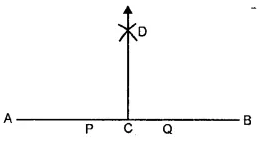2. With C as centre and any suitable radius draw a semi-circle to cut AB at P and
3. With P as centre and any radius more than PC draw an arc.
4. With Q as centre and same radius draw another arc to cut the previous arc at D.
5. Join CD. Then CD is the required line perpendicular to AB.
Verification : Measure ∠ACD. We see that ∠ACD = 90°.

Question 11.
Solution:
Steps of Construction :
1. With the help of rular, draw a line segment AB = 5.6 cm.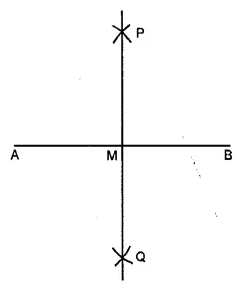2. With A as centre and radius more than half AB, draw arcs, one on each side of AB.
3. With B as centre and the same radius as before draw arcs, cutting the previous arcs at P and Q respectively.
4. Join PQ, meeting AB at M. Then, PQ is the required right bisector of AB.
Verification : On measuring AM and BM and ∠AMP, we see that AM = BM and ∠AMP = 90°.
So, PQ is the right bisector of AB.

Question 12.
Solution:
Steps of Construction :
1. With the help of a rular, draw a ray OA.
2. With O as centre and suitable radius draw an arc to cut OA at P.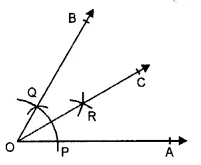3. With P as centre and the same radius, draw another are to cut the previous arc at Q.
4. Join OQ and produce it to any point B, then ∠AOB = 60° is the required angle.
5. With P as centre and radius more than half PQ, draw an arc.
6. With Q as centre and the same radius, draw another arc to cut the previous arc at R.
7. Join OR and produce it to the point C. Then OC is the required bisector of ∠AOB.
Verification : Measure ∠AOC and ∠BOC. We see that ∠AOC = ∠BOC. So, OC is the bisector of ∠AOB.

Question 13.
Solution:
Steps of construction :
1. Draw a ray OA with the help of a rular.
2. With O as centre and suitable radius draw an arc above OA to cut it at P.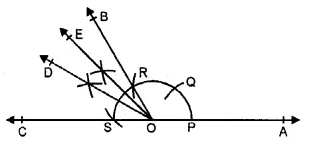3. With P as centre and same radius, cut the arc at Q and again with Q as centre and same radius cut the arc at R. With R as centre and same radius, again cut the arc at S.
4. Join OR and produce it to B and join OS and produce it to C.
5. Draw the bisector OD of ∠BOC.
6. Draw the bisector OE of ∠BOD. Then, ∠AOE = 135° is the required angle.

Hope given RS Aggarwal Solutions Class 6 Chapter 14 Constructions Ex 14A are helpful to complete your math homework.

If you have any doubts, please comment below. Learn Insta try to provide online math tutoring for you.